# Exponential Equations : Exponential Equations Quiz

Quiz
*Theme/Title: Exponential Equations
* Description/Instructions
The following equations can be solved for the variable without using logarithms. This can be done by making all of the base numbers equal by using the properties of powers including:

Zero Exponent Property: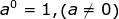Negative Exponent Property:Product of Powers Property: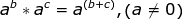Quotient of Powers Property:Power of a Product Property: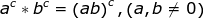Power of a Quotient Property: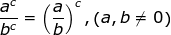Power of a Power Property: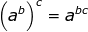Rational Exponent Property:Using the properties of powers, manipulate the following problems to contain a common base in order to solve for the variable.

For example: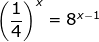Group: Algebra Algebra Quizzes
Topic: Exponential Equations